# On extensions of pseudo-valuations on BCK algebras

### Full PDFcreative_2022_31_1_43_49

Issue no:

In this paper we define a \textit{pseudo-valuation} on a BCK algebra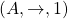as a real-valued function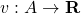satisfying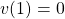and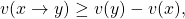for every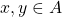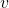is called a \textit{valuation} if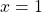whenever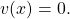We prove that every pseudo-valuation (valuation)induces a pseudo-metric (metric) ondefined by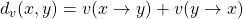for every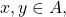where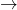is uniformly continuous in both variables. The aim of this paper is to provide several theorems on extensions of pseudo-valuations (valuations) on BCK algebras.